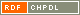# Show document

Title: Hamiltonian cycles in Cayley graphs whose order has few prime factors Kutnar, Klavdija (Author)Marušič, Dragan (Author)Morris, D. W. (Author)Morris, Joy (Author)Šparl, Primož (Author)http://amc.imfm.si/index.php/amc/article/view/177 English Not categorized 1.01 - Original Scientific Article IAM - Andrej Marušič Institute We prove that if Cay▫$(G; S)$▫ is a connected Cayley graph with ▫$n$▫ vertices, and the prime factorization of ▫$n$▫ is very small, then Cay▫$(G; S)$▫ has a hamiltonian cycle. More precisely, if ▫$p$▫, ▫$q$▫, and ▫$r$▫ are distinct primes, then ▫$n$▫ can be of the form kp with ▫$24 \ne k < 32$▫, or of the form ▫$kpq$▫ with ▫$k \le 5$▫, or of the form ▫$pqr$▫, or of the form ▫$kp^2$▫ with ▫$k \le 4$▫, or of the form ▫$kp^3$▫ with ▫$k \le 2$▫. graph theory, Cayley graphs, hamiltonian cycles 2012 str. 27-71 Vol. 5, no. 1 1855-3966 519.17 10243710281657 66Document is not linked to any category.

Average score: (0 votes) Voting is allowed only to logged in users.Hover the mouse pointer over a document title to show the abstract or click on the title to get all document metadata.

## Secondary language

Language: English teorija grafov, Cayleyjevi grafi, hamiltonski cikli